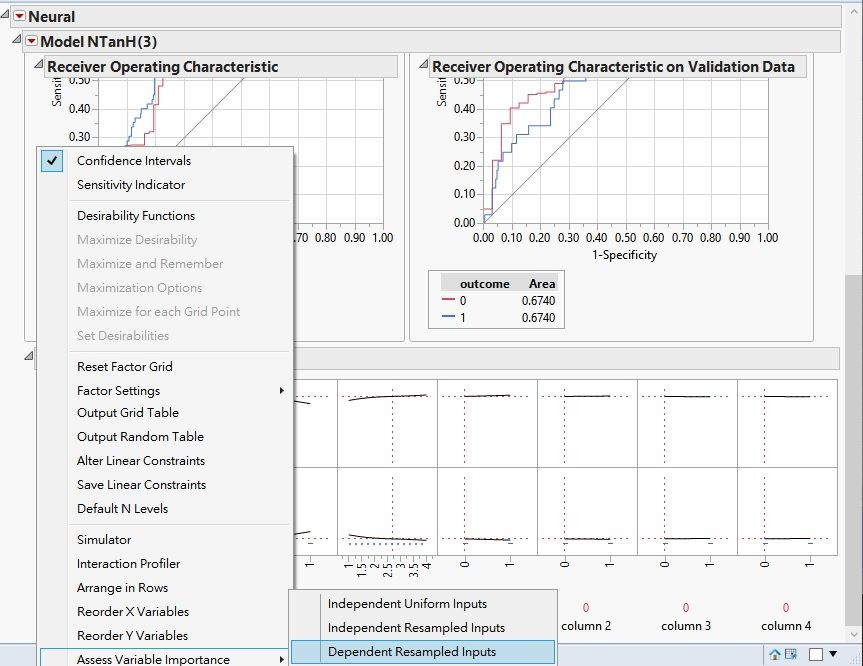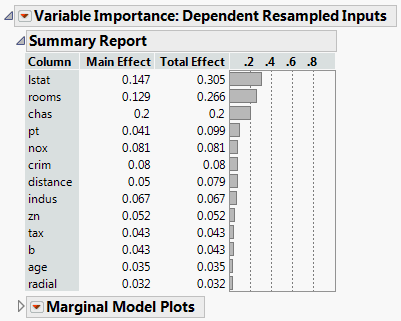World Statistics Day was yesterday, but we’re celebrating all week long! This celebration means acknowledging the impact statistics has on our world. Who is your favorite statistician? Share with us who they are and why they top your favorites list.
Choose Language Hide Translation Bar
Highlighted

## What is k when assessing variable importance?

Hi JMP Community, I was running neural networks to construct prediction models by JMP and assessing variable importance by Dependent Resampled Inputs, which using a k-nearest neighbors approach.  I had the variable importance for each variable in the model, but how can I know the value of k and the other details of the k-nearest neighbors approach?

1 ACCEPTED SOLUTION

Accepted Solutions
Highlighted

## Re: What is k when assessing variable importance?

I do not think that the importance result is based on k. The help system says:

Factor values are constructed from observed combinations
using a k-nearest neighbors approach, in order to account for correlation. This option
treats observed variance and covariance as representative of the covariance structure for
your factors. Use this option when you believe that your factors are correlated. Note that
this option is sensitive to the number of rows in the data table. If used with a small number
of rows, the results can be unreliable.

Further:

Note: Variable importance indices are constructed using Monte Carlo sampling. For this reason, you can expect some variation in importance index values from one run to another.

In other words, a k-nearest neighbors approach is used to cluster observations so that the covariance structure of the data can be maintained. These observations are put into the model to get predicted values. Now, repeat (this is the Monte Carlo part) by choosing a new set of observations. See how much a change in a single factor made so that you can assess the importance of that variable.

I do not know all of the details of the k-nearest neighbors approach that is used, but I would guess that if a choice of k is made, it would be the one that describes the data the best. Look at the k-nearest neighbors clustering technique in the help section to see how JMP "optimally" chooses a k in that situation. If this approach is truly followed, I do not know what range of k is explored. Either way, I would bet that a range of k values are used due to the monte carlo simulation that is going on.

Dan Obermiller
4 REPLIES 4
Highlighted

## Re: What is k when assessing variable importance?

Can u share a quick screenshot of what you're asking?
Highlighted

## Re: What is k when assessing variable importance?

Thank you for replying. I did prediction model of neural network, I selected Profilers>Assess Variable Importance>Dependent Resampled Inputs, and will show a list of variable importance. According to the JMP Help, the importance was calculated using a k-nearest neighbors approach. My question is that if I can know the k value of this k-nearest neighbors approach?

Highlighted

## Re: What is k when assessing variable importance?

Thank you for replying. I did prediction model of neural network, I selected Profilers>Assess Variable Importance>Dependent Resampled Inputs, and will show a list of variable importance. According to the JMP Help, the importance was calculated using a k-nearest neighbors approach. My question is that if I can know the k value of this k-nearest neighbors approach?Highlighted

## Re: What is k when assessing variable importance?

I do not think that the importance result is based on k. The help system says:

Factor values are constructed from observed combinations
using a k-nearest neighbors approach, in order to account for correlation. This option
treats observed variance and covariance as representative of the covariance structure for
your factors. Use this option when you believe that your factors are correlated. Note that
this option is sensitive to the number of rows in the data table. If used with a small number
of rows, the results can be unreliable.

Further:

Note: Variable importance indices are constructed using Monte Carlo sampling. For this reason, you can expect some variation in importance index values from one run to another.

In other words, a k-nearest neighbors approach is used to cluster observations so that the covariance structure of the data can be maintained. These observations are put into the model to get predicted values. Now, repeat (this is the Monte Carlo part) by choosing a new set of observations. See how much a change in a single factor made so that you can assess the importance of that variable.

I do not know all of the details of the k-nearest neighbors approach that is used, but I would guess that if a choice of k is made, it would be the one that describes the data the best. Look at the k-nearest neighbors clustering technique in the help section to see how JMP "optimally" chooses a k in that situation. If this approach is truly followed, I do not know what range of k is explored. Either way, I would bet that a range of k values are used due to the monte carlo simulation that is going on.

Dan Obermiller
Article Labels